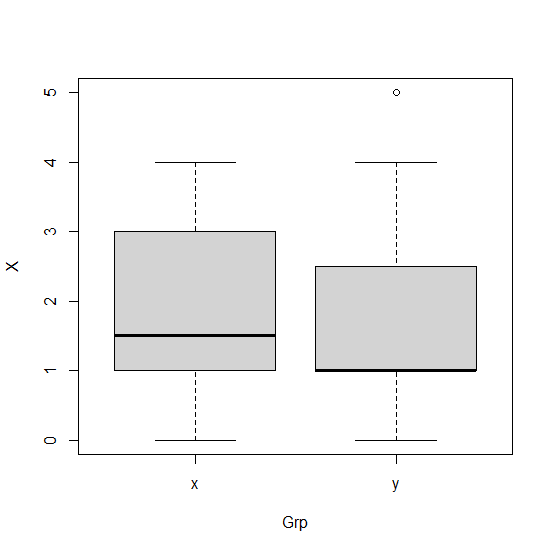# How to create boxplot of vectors having different lengths in R?

If we have multiple vectors of different lengths then the boxplot for such vectors can be created by creating a single data frame using those vectors with a categorical column showing the name of the vectors and a numerical column having the corresponding values. Then boxplot function will be used as shown in the below example.

## Example

Consider the below vector x and y and create the data frame using them −

Live Demo

> x<-rpois(20,2)
> y<-rpois(15,2)
> df<-data.frame(X=c(x,y),Grp=rep(c("x","y"),times=c(20,15)))
> df

## Output

   X Grp
1  4   x
2  2   x
3  1   x
4  2   x
5  0   x
6  2   x
7  3   x
8  1   x
9  0   x
10 1   x
11 3   x
12 4   x
13 2   x
14 3   x
15 4   x
16 1   x
17 1   x
18 1   x
19 1   x
20 1   x
21 1   y
22 0   y
23 1   y
24 4   y
25 1   y
26 1   y
27 2   y
28 3   y
29 1   y
30 5   y
31 2   y
32 0   y
33 1   y
34 4   y
35 1   y

Creating the boxplot for groups in df −

> boxplot(X~Grp,data=df)

## Output